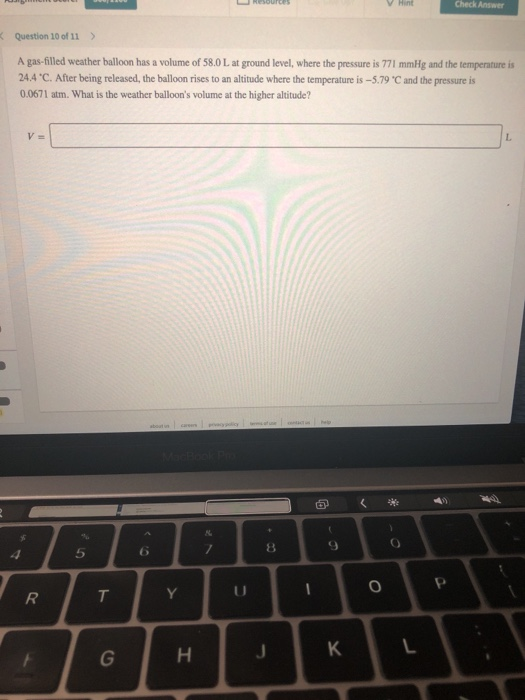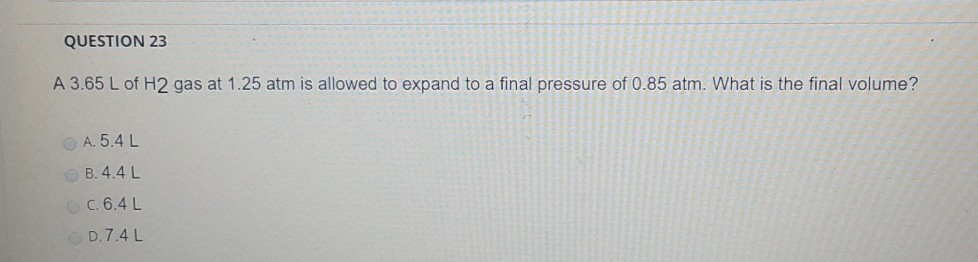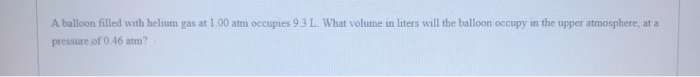Question

# A gas-filled balloon having a volume of 2.90 L at 1.25 atm and 23 degrees Celsius...

A gas-filled balloon having a volume of 2.90 L at 1.25 atm and 23 degrees Celsius is allowed to rise to the stratosphere (about 30 km above the surface of the Earth), where the temperature and pressure are -44 degrees Celsius and 5.30 x 10-3 atm, respectively. Calculate the final volume of the balloon.

By using combined gas law

P1V1/T1 = P2V2/T2

P1= initial pressure = 1.25 atm ; P2 = final pressure = 5.30*10^-3 atm

V1= initial volume = 2.90 L ; V2 = final volume = ?

T1= initial temperature = 23+273.15= 296.15 K

T2= final temperature = -44+273.15= 229.15 K

V2= P1V1T2/T1P2

= (1.25*2.90*229.15)/(296.15*5.30*10^-3)

529.2 L

#### Earn Coins

Coins can be redeemed for fabulous gifts.

Similar Homework Help Questions
• ### A gas-filled balloon having a volume of 2.70 L at 1.20 atm and 26 ° C...

A gas-filled balloon having a volume of 2.70 L at 1.20 atm and 26 ° C is allowed to rise to the stratosphere (about 30 km above the surface of the Earth), where the temperature and pressure are −41 ° C and 5.90 × 10−3 atm, respectively. Calculate the final volume of the balloon.

• ### A gas-filled balloon having a volume of 3.60 L at 1.10 atm and 24°C is allowed...

A gas-filled balloon having a volume of 3.60 L at 1.10 atm and 24°C is allowed to rise to the stratosphere (about 30 km above the surface of the Earth), where the temperature and pressure are −53°C and 4.80 × 10−3 atm, respectively. Calculate the final volume of the balloon.

• ### A gas-filled balloon with a volume of 2.10 L at 1.20 atm and 20°C is allowed...

A gas-filled balloon with a volume of 2.10 L at 1.20 atm and 20°C is allowed to rise to the stratosphere (about 30 km above the surface of the Earth), where the temperature and pressure are −23°C and 3.00×10−3 atm, respectively. Calculate the final volume of the balloon.

• ### A sample of N2 gas has a volume of 25.0 L at a pressure of 1.50 atm and a temperature of 23 degrees Celsius. What volum...

A sample of N2 gas has a volume of 25.0 L at a pressure of 1.50 atm and a temperature of 23 degrees Celsius. What volume, in liters, will the gas occupy at 3.50 atm and 251 degrees Celsius? Assume ideal behavior. V= L

• ### Question 10 of 11 > A gas-filled weather balloon has a volume of 58.0 L at...Question 10 of 11 > A gas-filled weather balloon has a volume of 58.0 L at ground level, where the pressure is 771 mmHg and the temperature is 24.4 "C. After being released, the balloon rises to an altitude where the temperature is -5.79 "Cand the pressure is 0.0671 atm. What is the weather balloon's volume at the higher altitude? Truop

• ### QUESTION 23 A 3.65 L of H2 gas at 1.25 atm is allowed to expand to...QUESTION 23 A 3.65 L of H2 gas at 1.25 atm is allowed to expand to a final pressure of 0.85 atm. What is the final volume? A. 5.4L B. 4.4L c. 6.4L D.7.4L

• ### a) A helium-filled weather balloon has a volume of 521 L at 17.9°C and 758 mmHg....

a) A helium-filled weather balloon has a volume of 521 L at 17.9°C and 758 mmHg. It is released and rises to an altitude of 5.22 km, where the pressure is 472 mmHg and the temperature is –14.1°C. The volume of the balloon at this altitude is ______L b) A sample of krypton gas occupies a volume of 6.19 L at 56.0°C and 382 torr. If the volume of the gas sample is decreased to 4.90 L, while its temperature...

• ### A balloon filled with helium gas at 1.00 atm occupies 9.3 L. What volume in liters...A balloon filled with helium gas at 1.00 atm occupies 9.3 L. What volume in liters will the balloon occupy in the upper atmosphere, at a pressure of 0.46 atm?

• ### 1. A helium-filled weather balloon has a volume of 558 L at 22.9°C and 751 mmHg....

1. A helium-filled weather balloon has a volume of 558 L at 22.9°C and 751 mmHg. It is released and rises to an altitude of 3.13 km, where the pressure is 582 mmHg and the temperature is 1.9°C. The volume of the balloon at this altitude is ____L. 2. A sample of helium gas collected at a pressure of 1.42 atm and a temperature of 17.0 °C is found to occupy a volume of 22.9 liters. How many moles of...

• ### If gas at STP in a 1.05 L balloon is heated to 50 degrees C while...

If gas at STP in a 1.05 L balloon is heated to 50 degrees C while the volume is allowed to rise to 2.00 L, what pressure will the gas be exerting on the balloon?